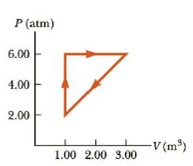Chapter 12, Problem 31P

Chapter
Section
Textbook Problem

A gas increases in pressure from 2.00 atm to 6.00 atm at a constant volume of 1.00 m3 and then expands at constant pressure to a volume of 3.00 m3 before returning to its initial state as shown in Figure P12.31. How much work is done in one cycle?Figure P12.31

To determine
the total work done in one cycle.

Explanation

Given Info:

The initial pressure of the gas is 2atm .

The final pressure of the gas is 6atm .

The initial volume of the gas is 1.00m3 .

The initial volume of the gas is 3.00m3 .

The total work done by a heat engine operating on a cycle is the area enclosed by the curve in PV diagram.

Formula to calculate the area enclosed by the curve in PV diagram is,

A=12(B×H)

• B is the base of the triangle
• H  is the height of the triangle

Substitute 4atm for B and 2m3<

Still sussing out bartleby?

Check out a sample textbook solution.

See a sample solution

The Solution to Your Study Problems

Bartleby provides explanations to thousands of textbook problems written by our experts, many with advanced degrees!

Get Started